# The optimal solution

Suppose we’ve got the Tower of Hanoi puzzle with three pegs and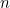discs. We will show that for the usual starting and finishing configurations of discs, all discs sitting on one peg, the shortest solution involves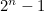moves. For any other pair of starting configurations the shortest solution involves at mostmoves.

First let’s consider the game with one disc. Clearly solving the puzzle only involves one move. Since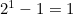the result is true for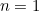.

Now suppose you’re playing the game with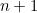discs using the usual starting and finishing configurations and assume that the result is true for the-disc version of the game: the smallest number of moves required is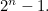To solve the-disc version, first move thesmaller discs onto one of the free pegs using the shortest method which takesmoves. Now move the largest disc onto the remaining free peg. Then shift the-tower on top of it, again using the method that takesmoves. You’ve now solved the puzzle in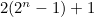moves. It’s easy to see that this is the shortest solution as you cannot get away from moving the-tower at least twice and the largest disc at least once. Therefore, the shortest number of moves required to solve the-disc version of the game is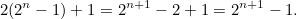This shows that if the result is true forit is true for. So we have proved by induction that the result is true for all.

Now suppose you are playing the game with arbitrary starting and finishing configurations of discs. We will show that at mostmoves are required to solve the puzzle.

Again we start with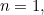in which case there are only three possible configurations separated pairwise by one move. So the result is true.

Now assume it is true forand see what happens for.

In the Hanoi graph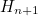writeand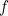for the nodes representing the starting and finishing configurations respectively and write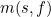for the length of the shortest path taking you fromto. (By the length of the path we mean the number of edges it traverses.) The Hanoi graphconsists of three copies of the Hanoi graph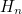joint pairwise by one edge. Ifandlie within the same copy ofthen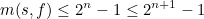by our hypothesis that the result is true for. Suppose they lie in two different copies, copy 1 and copy 2 of. You can get fromtoby moving fromto the vertex in copy 1 that is linked to copy 2, traversing the linking edge and then moving towithin copy 2. By our assumption that the result is true forthe first and the last part of this route have length at most. Thus,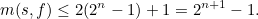QED

Back to main article

• Want facts and want them fast? Our Maths in a minute series explores key mathematical concepts in just a few words.